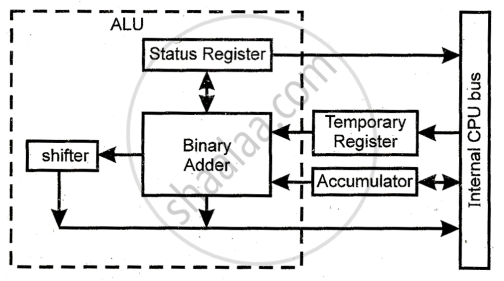HSC Science (Computer Science) 12th Board ExamMaharashtra State Board
Share

# Explain the Function of Alu with a Simple Block Diagram. - HSC Science (Computer Science) 12th Board Exam - Computer Science 2

ConceptIntroduction to Microprocessors and Organization of 8085

#### Question

Explain the function of ALU with a simple block diagram.

#### Solution

The organization of arithmetic and logic unit is shown in the figure.1) The arithmetic and logic unit is an 8-bit unit.

2) It performs arithmetic, logic and rotates operations.

3) It consists of the binary adder to perform addition and subtraction by 2’s complement method.

4) The result is typically stored in an accumulator.

5) Accumulator, temporary register and flag register are closely associated with A.L.U.

6) The temporary register is used to hold data during an arithmetic/ logic operation.

7) The flags are set or reset according to the result of operations in the status register.

Is there an error in this question or solution?

#### APPEARS IN

2017-2018 (March) (with solutions)
Question 4.1.2 | 3.00 marks
Solution Explain the Function of Alu with a Simple Block Diagram. Concept: Introduction to Microprocessors and Organization of 8085.
S